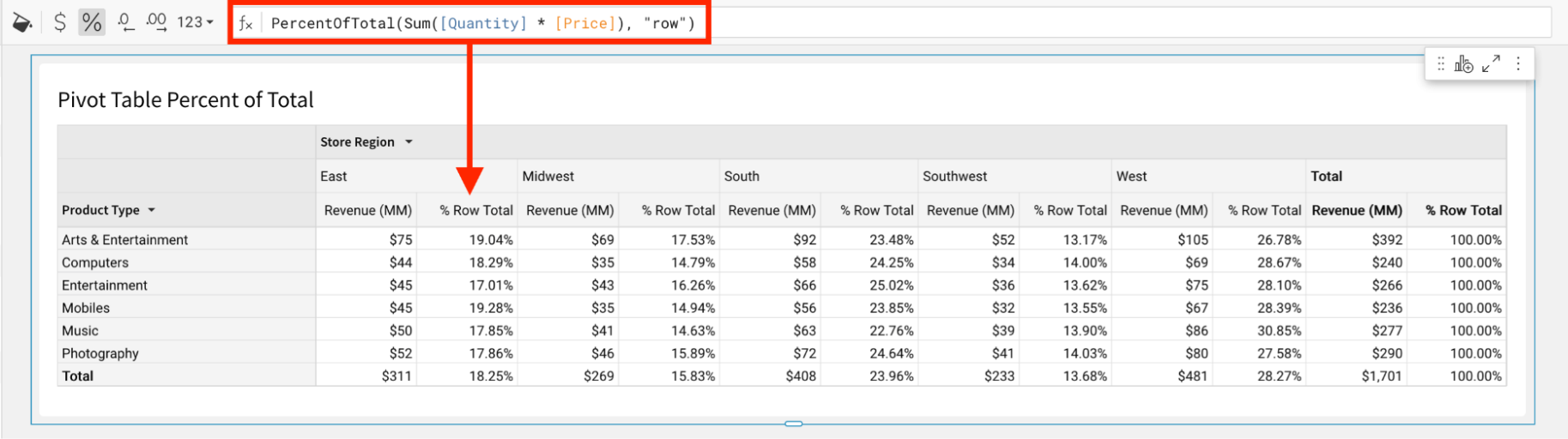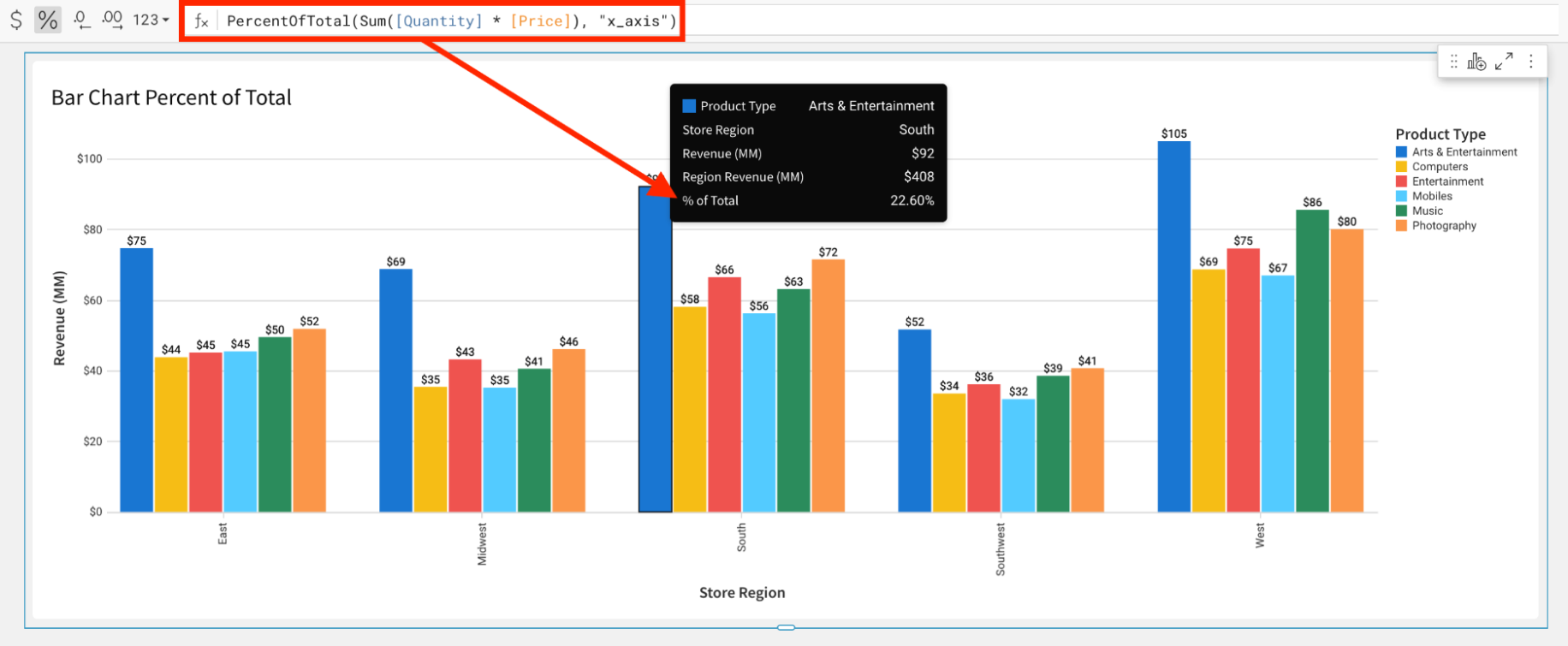# PercentOfTotal

The PercentOfTotal function returns the percent of total for an aggregate formula.

This function is shorthand for: aggregate / Subtotal(aggregate, mode, parameters).

## Syntax

`PercentOfTotal(aggregate, [mode], [parameters])`

Function arguments:

• aggregate (required) - The aggregate formula to use
• mode (optional) - The mode to use when calculating the aggregate formula. Mode determines which dimension(s) to use when performing the calculation.
• General purpose modes
• `“grand_total”` (default for visualizations and pivot tables) - Calculates the percent of grand total for the aggregate formula.
• Visualization modes
• `“color”` - Calculates the aggregate percent of total using only the column specified for COLOR.
• `“x_axis”` - Calculates the aggregate percent of total using only the column(s) placed on the X-AXIS.
• `“trellis_column”` / `“trellis_row”` -Calculates the aggregate percent of total using only the dimension specified for the TRELLIS COLUMN or TRELLIS ROW.
• Pivot table modes
• `“column”` / `“row”` - Calculates aggregate percent of total for the column or row total.
• `“column_parent”` / `“row_parent”` - Calculates the aggregate percent of total for the column or row total, relative to the subtotal calculation for the parent dimensions.
• Table modes
• `“grouping_parent”` (default for tables) - Calculates the aggregate formula using the grouping keys from a parent grouping. This is equivalent to creating the aggregate calculation in a parent grouping and referencing it from a column in a lower grouping.
• parameters (optional) - An additional parameter specific to the mode.
• Only applicable to the following modes: "column_parent", "row_parent" and "grouping_parent". For these modes, this 3rd parameter specifies how many parent dimensions to ignore.

## Examples

`PercentOfTotal(Sum([Quantity] * [Price]), "row")`
• The numerator of the percentage is the aggregate formula calculated along rows and columns.
• The denominator of the percentage is the aggregate formula calculated along the rows.
• The pivot table subtotals calculate the percent of total at the expected granularity.`PercentOfTotal(Sum([Quantity] * [Price]), "x_axis")`
• The numerator of the percentage is the aggregate formula calculated along the x-axis and color.
• The denominator of the percentage is the aggregate formula calculated along the x-axis.
• The calculation is shown in the tooltip, but is usable anywhere an aggregate formula is allowed.